# Potential Divider

A potential divider is widely used in circuits. It is based on the principle that the potential drop across a segment of a wire of uniform cross-section carrying a constant current is directly proportional to its length. It is used in the volume control knob of music systems.  Sensory circuits using light-dependent resistors and thermistors also use potential dividers.

Potential Divider

A potential divider consists of two resistors (R1 and R2) in series. The current I through both the resistors is the same. The potential across resistor R1 is V1 and R2 is V2. The potential difference across the resistors can be mathematically written using Ohm’s law.Figure 1: Potential DividerUsing the above equation, it can be understood that the total potential difference (V) is divided between the two resistors according the ratio of their resistances. By choosing the appropriate resistor values, the potential difference across the resistances can be varied.

Resistance

The resistance of a uniform conductor depends upon:

• Its length, l
• Its cross-sectional area, A
• The resistivity of the material, ρ

The resistivity is different for different materials and varies greatly with temperature. It is measured in Ω m.

The relationship between resistance and the three quantities can be represented using mathematical equation as given below.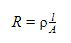From the above equation of resistance, it can be noted that the value of resistance increases with increase in the length of the conductor.

Variable Resistor

A variable resistor (rheostat) can be used to control current in a circuit. A variable resistor consists of a length of resistance wire and an adjustable sliding contact. Without switching off the circuit, the resistance can be varied using a sliding contact. The symbol for a variable resistor is given in the diagram below.Figure 2: Circuit Symbol of Variable Resistor

A rheostat is made using a resistance wire, which is wound around circular insulation. A sliding contact is placed in the wire to change the length of the resistor. An end of the wire and sliding contact is connected to the circuit. As the length of the resistance wire is changed, the resistance also changes. The resistance can be set to any value from nearly zero to the total resistance of the wire in the variable resistor. A rheostat is used in car lighting systems to change the brightness of the lights.

Potentiometer

The design of a potentiometer is similar to that of a variable resistor. All the three points, both the ends of resistance wire and the adjustable contact, are connected to the circuit.Figure 3: Circuit Symbol for Potentiometer

Two terminals and the contact are connected to the circuit. The length of the wire can be changed by the sliding contact. The resistance increases as the length of the wire increases. The resistance can be set to any value from zero to the total resistance of the wire. Potentiometers are often used, for example, to change the volume in a speaker system.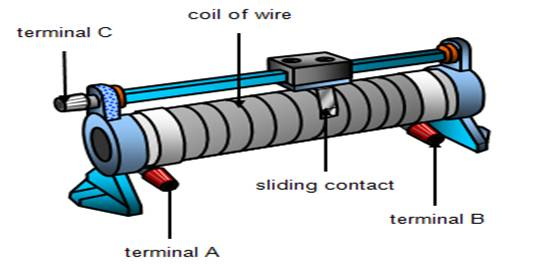Figure 4: A Potentiometer

Application of Potential Dividers

Potential dividers are widely used in sensory circuits. The change in the physical property of a sensor has to be processed before it can be displayed or measured. Light-dependent resistors and thermistors are two examples of sensory devices whose resistances vary with light and temperature respectively. The resistance of a light-dependent resistor decreases as the light intensity increases. The resistance of the thermistor decreases with rise in temperature. A potential divider can be used to process the information obtained from these sensory devices.

Let us consider a potential divider circuit as shown in Figure 1. A sensory device can be placed in the position of R2.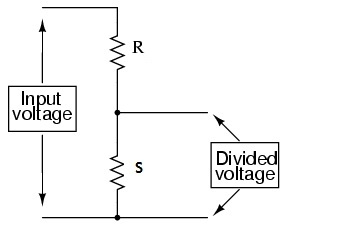Figure 5: Potential Divider in Sensory Circuits

The voltage across sensory device (Vs) can be mathematically written as: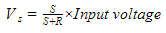The magnitude of Vs depends on the relative resistance of R and S. We can note that, as the resistance of sensory device (S) increases, the voltage also increases.

Example

A potential divider circuit can be used inside a refrigerator to switch on the cooling circuit when the temperature is high (more than 3°C).Figure 6: Potential Divider Using a Thermistor

The characteristics of the thermistor are given in the table below. Let the voltage across the cooling circuit be VCC and the resistance of cooling circuit is 5kΩ. In order for the cooling circuit to operate, it needs a potential difference of 5 V or more.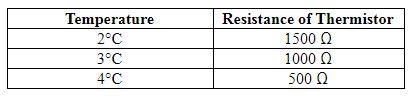Hence, this potential divider meets the requirements of the refrigerator. These circuits can be further modified to suit different applications. For example: switching off a heater when the temperature is above a certain temperature. This circuit can also be used for switching off lights in the daytime and switching them on at night (using LDR).

Summary

• Two resistors in series act as a potential divider, where• If V is the input voltage, the divided voltage across the output can be given by the equation• A potentiometer is a variable resistor connected as a potential divider to give a continuously variable output voltage.